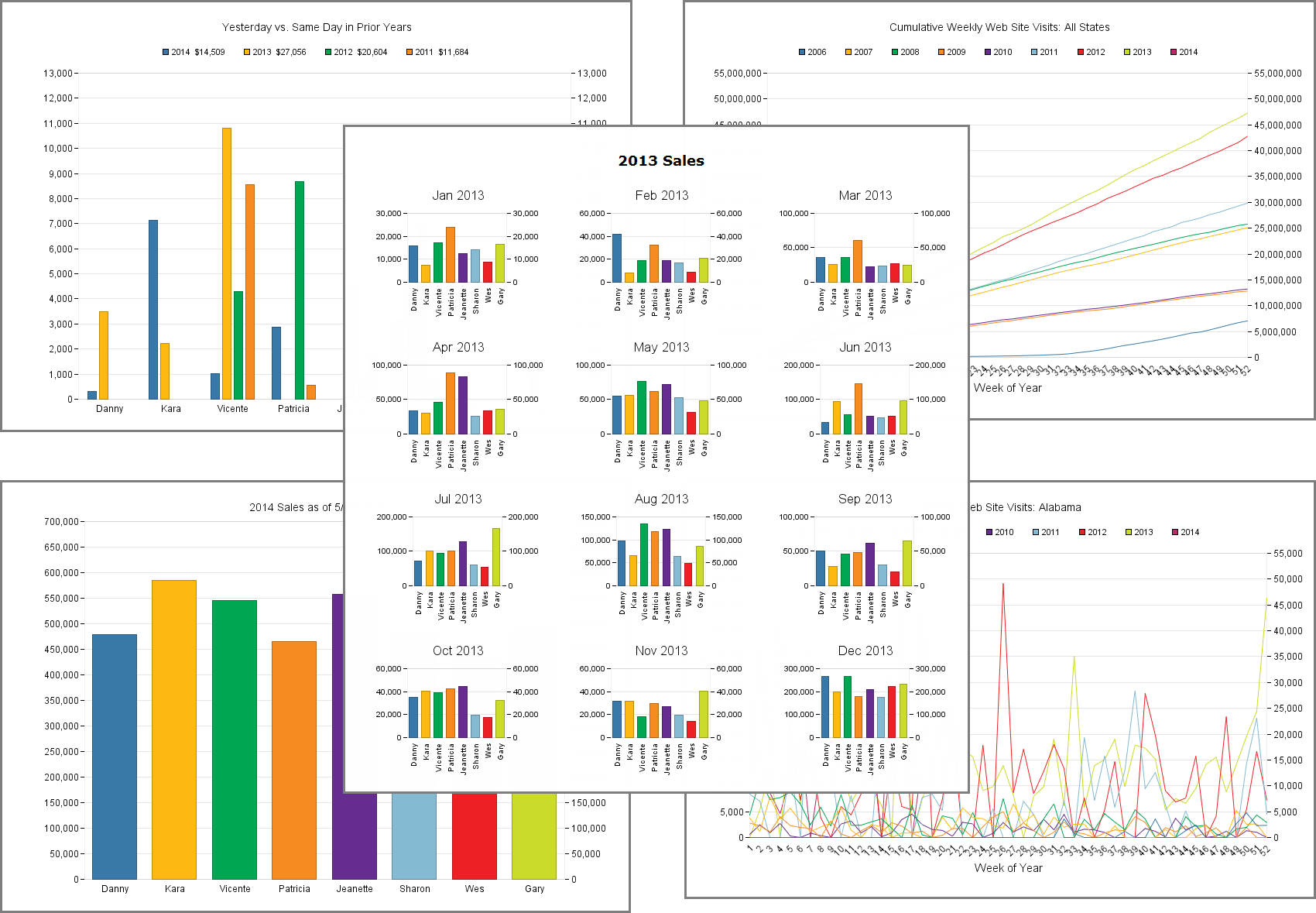## FM 13: Virtual List Charts, part 2

Picking up where we left off in part 1, today we’re going to take a look at examples 2 through 6 in the Virtual List Charts demo file (the demo has been updated since part 1, so I recommend downloading a fresh copy).We covered example 1 and most of the general concepts last time, so today we’re mainly going to touch on specific points of interest, but to briefly recap… Continue reading “FM 13: Virtual List Charts, part 2”

## The Last Day of the Month, part 3

Grizzled FileMaker veterans are fond of saying things like, “You ought to know at least three different ways to accomplish any given task.” With that in mind, I hereby submit a third method for calculating the last day of a given month.

``` Let ( [ theDate = Get ( CurrentDate ) ; monthNum = Month ( theDate ) ; yearNum = Year ( theDate ) ; febLastDay = 28 + Case ( Mod ( yearNum ; 400 ) = 0 ; 1 ; Mod ( yearNum ; 100 ) = 0 ; 0 ; Mod ( yearNum ; 4 ) = 0 ; 1 ; 0 ) ; dayNum = Choose ( monthNum ; "" ; 31 ; febLastDay ; 31 ; 30 ; 31 ; 30 ; 31 ; 31 ; 30 ; 31 ; 30 ; 31 ) ] ;```

``` Date ( monthNum ; dayNum ; yearNum ) ```

`) // end let`

If you read yesterday’s post, you may have noted a resemblance between this calculation and its predecessor, at least as far as the “Let” portion goes. The main difference is the addition of a dayNum variable populated via the Choose() function.

In case you’re not comfortable with Choose(), its format is

``` Choose ( test ; result if test = 0 { ; result if test = 1 ; result if test = 2... } )```

…where “test” is any non-negative whole number, and the results in braces are optional. At first this function may seem confusing but it turns out to be a very compact replacement for the Case() function, under a strictly defined set of circumstances.

Say, for example, in a table called “test”, you have a field called “score”, which can contain any integer between 0 and 9, and you want convert that value to its corresponding name (“zero,” “one,” “two”, etc.). You could certainly accomplish this with Case() and the statement might look like this:

``` Case ( test::score = 0 ; "zero" ; test::score = 1 ; "one" ; test::score = 2 ; "two" ; test::score = 3 ; "three" ; test::score = 4 ; "four" ; test::score = 5 ; "five" ; test::score = 6 ; "six" ; test::score = 7 ; "seven" ; test::score = 8 ; "eight" ; test::score = 9 ; "nine" )```

The exact same result can be obtained far more economically thus:

``` Choose ( test::score ; "zero" ; "one" ; "two" ; "three" ; "four" ; "five" ; "six" ; "seven" ; "eight" ; "nine" )```

Essentially, Choose uses test::score as a pointer to the correct “result”, via what’s known as a zero-based index, so a test::score value of 0 corresponds to the first result, a test::score of 1 corresponds to the second result, etc.

In the case of our Last Day of the Month problem, there is no month number of 0, only 1 through 12, so our first result is `""` to accommodate the non-existent zero result.